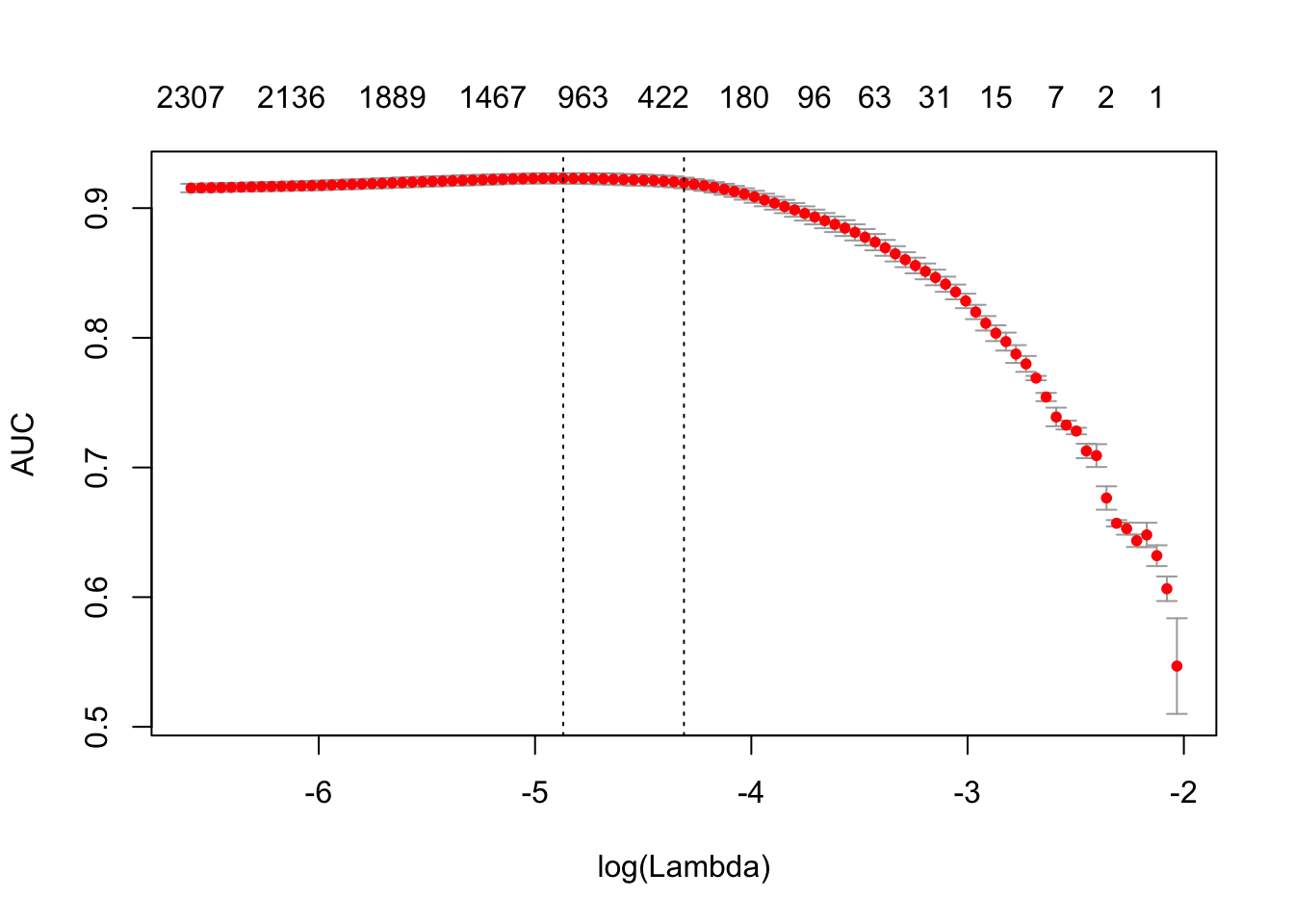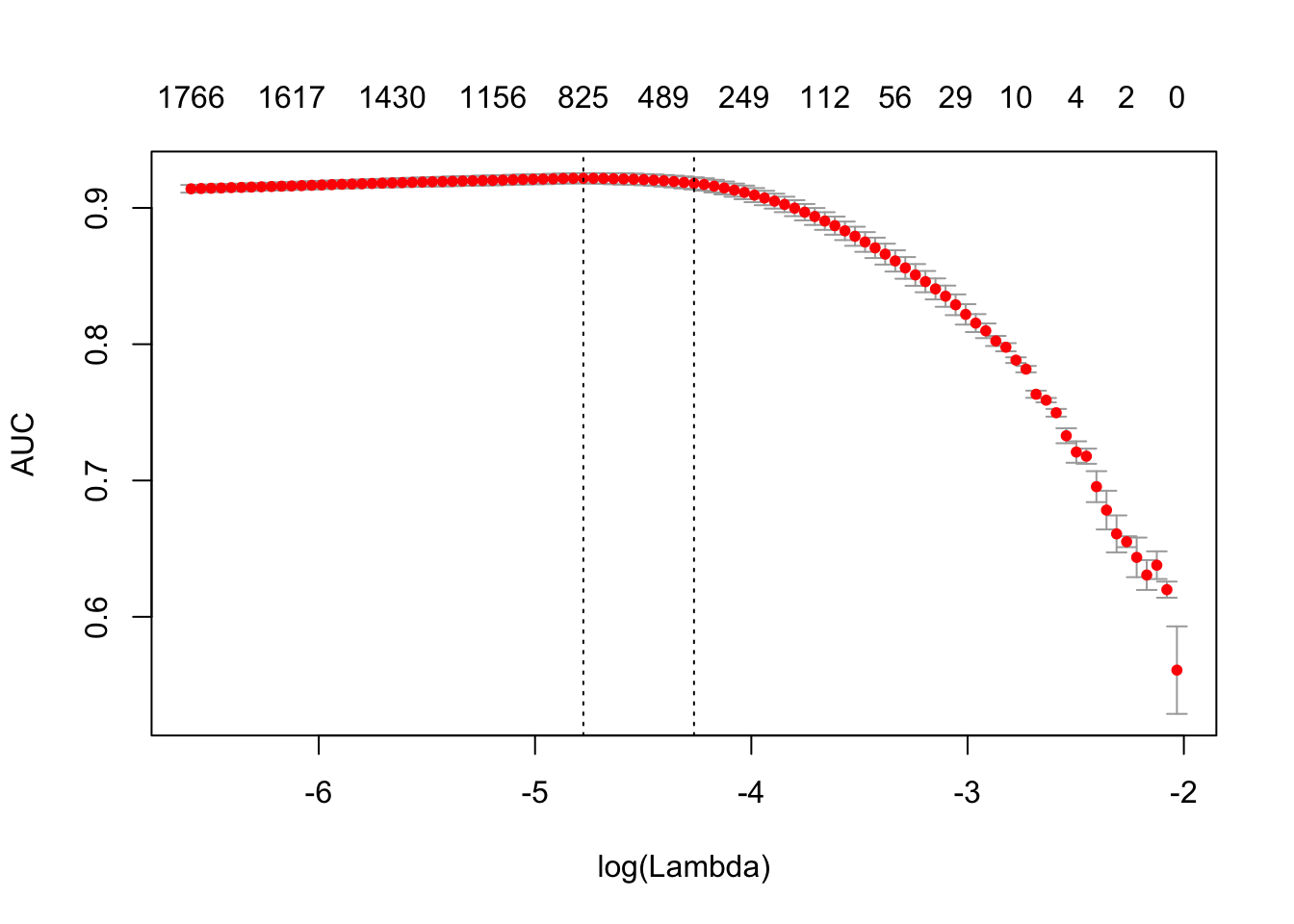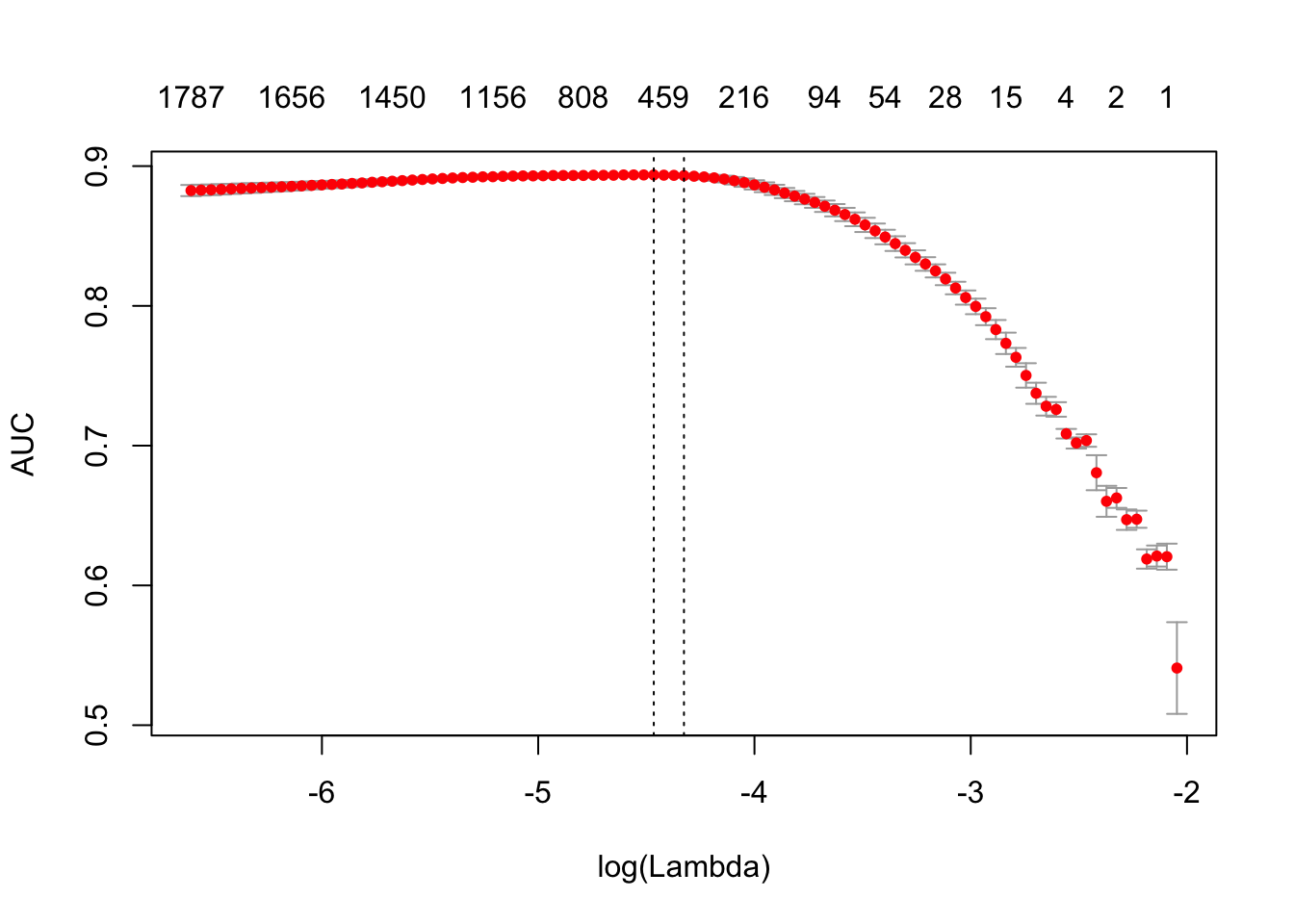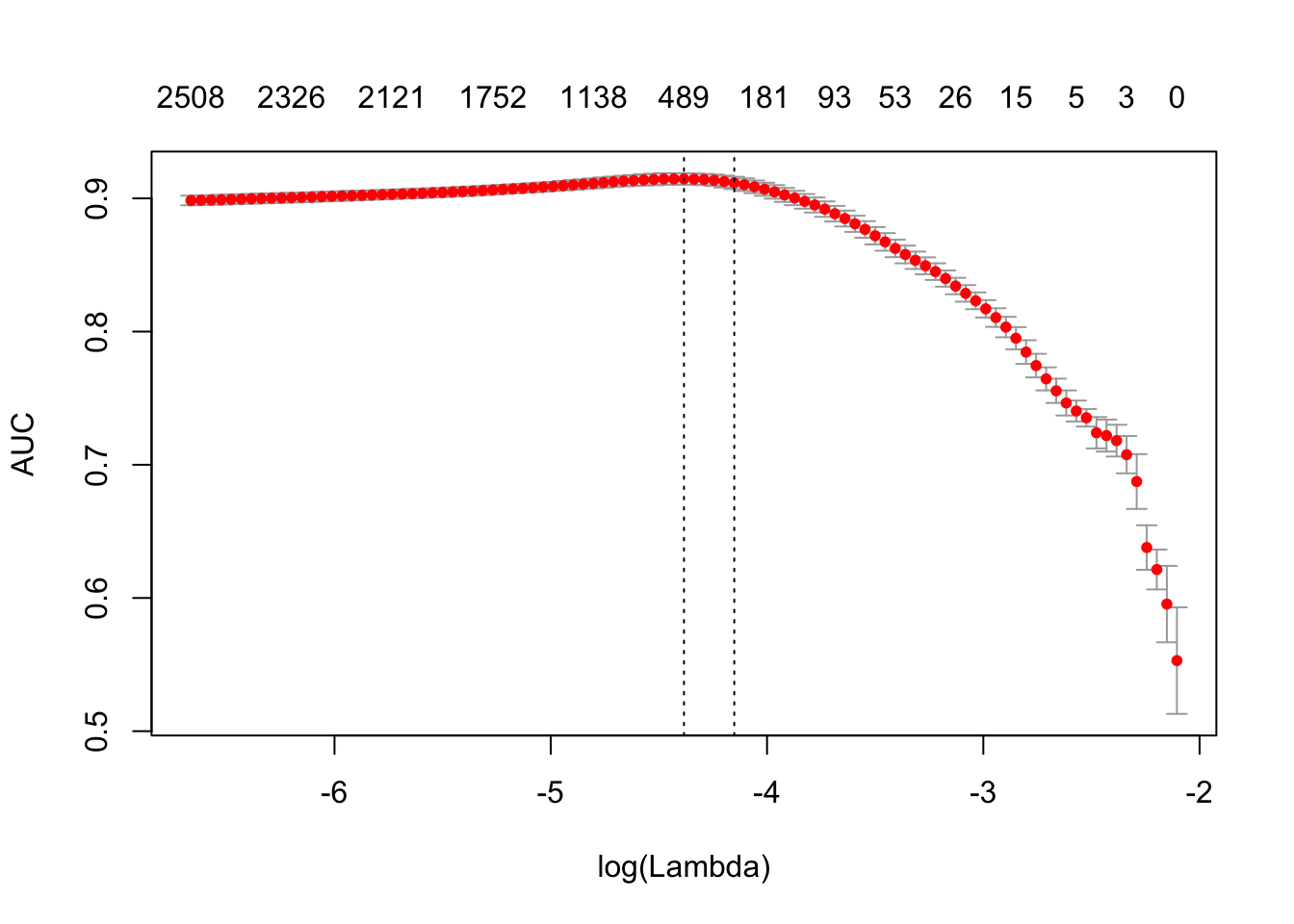# 文本分析工作流

1. 研究者从输入文本构建 document-term matrix (DTM) 或者 term-co-occurrence matrix (TCM)。或者说，第一步是构建一个映射，将词或者 n-gram 映射到一个向量空间（vector space）

2. 研究者拟合 DTM 模型，这些模型可以是文本分类模型，主题模型，相似性研究等等。拟合模型的过程包括调优和验证模型。

3. 最后研究者将模型运用在新数据上。

text2vec 包提供了一个比上述方法更好的解决方案来处理 document-term matrix。

text2vec 包提供了 movie_review 数据集，它包含 5000 个电影评论，每个评论被标记为正面或者负面。我们将会使用 data.table 包来预处理数据。

library(text2vec)
library(data.table)
data("movie_review")
setDT(movie_review)
setkey(movie_review, id)
set.seed(2016L)
all_ids = movie_review$id train_ids = sample(all_ids, 4000) test_ids = setdiff(all_ids, train_ids) train = movie_review[J(train_ids)] test = movie_review[J(test_ids)] # 向量化 为了在向量空间里面展示文档，我们首先需要生成多个字段（terms）到字段（term）的 IDS。我们把他们叫做字段而不是词（words），是因为他们可以是任意 n-gram 而不仅仅是单个词。我们将一个集合的文档表示为稀疏矩阵，每一行代表一个文档，每一列代表一个 term。可以用两种方法来处理文本，使用词汇表本身，或者使用 feature hashing ## 基于词汇表的向量表 让我们先来生成一个基于词汇表的 DTM。我们从所有文档中收集独立的字段，使用 create_vocabulary() 将每一个词用一个独立的ID 来进行标记。 # 定义预处理函数以及 tokenization 函数 prep_fun = tolower tok_fun = word_tokenizer it_train = itoken(train$review,
preprocessor = prep_fun,
tokenizer = tok_fun,
ids = train$id, progressbar = FALSE) vocab = create_vocabulary(it_train) 这里完成了什么？ 1. 使用 itoken() 对 tokens 生成一个迭代器。所有包含 create_ 前缀的函数都能处理这些迭代区。R 用户可能会觉得这类模型不是很常见，但是迭代器允许我们隐藏输入的大部分的细节，并以内存友好的方式来处理数据。 2. 我们使用 create_vocabulary() 函数构建一个词汇表。 或者我们能够生成一列 tokens，然后在后续的步骤中重用他们。列表里面的每个元素代表一个文档，每个元素为一个包含 tokens 的文本向量。 train_tokens = train$review %>%
prep_fun %>%
tok_fun
it_train = itoken(train_tokens,
ids = train$id, # turn off progressbar because it won't look nice in rmd progressbar = FALSE) vocab = create_vocabulary(it_train) vocab ## Number of docs: 4000 ## 0 stopwords: ... ## ngram_min = 1; ngram_max = 1 ## Vocabulary: ## terms terms_counts doc_counts ## 1: overturned 1 1 ## 2: disintegration 1 1 ## 3: vachon 1 1 ## 4: interfered 1 1 ## 5: michonoku 1 1 ## --- ## 35592: penises 2 2 ## 35593: arabian 1 1 ## 35594: personal 102 94 ## 35595: end 921 743 ## 35596: address 10 10 注意到 text2vec 提供了一些 tokenizer 函数（见?tokenizers），这些函数是 base::gsub() 的简单接口，他们的速度不是很快，如果你需要更加智能或者更快速的包，可以使用 tokenizers。这个包可以满足绝大多数多使用需求。你还可以使用 stringi 包来写你自己多 tokenizer。 现在我们有了一个词汇表，我们接着来建立一个 document-term matrix。 vectorizer = vocab_vectorizer(vocab) t1 = Sys.time() dtm_train = create_dtm(it_train, vectorizer) print(difftime(Sys.time(), t1, units = 'sec')) ## Time difference of 0.8716209 secs 现在我们有了一个 DTM，我们可以查看他的维度。 dim(dtm_train) ##  4000 35596 identical(rownames(dtm_train), train$id)
##  TRUE

library(glmnet)
NFOLDS = 4
t1 = Sys.time()
glmnet_classifier = cv.glmnet(x = dtm_train, y = train[['sentiment']],
family = 'binomial',
# L1 penalty
alpha = 1,
# interested in the area under ROC curve
type.measure = "auc",
# 5-fold cross-validation
nfolds = NFOLDS,
# high value is less accurate, but has faster training
thresh = 1e-3,
# again lower number of iterations for faster training
maxit = 1e3)
print(difftime(Sys.time(), t1, units = 'sec'))
## Time difference of 2.747643 secs
plot(glmnet_classifier)print(paste("max AUC =", round(max(glmnet_classifier$cvm), 4))) ##  "max AUC = 0.923" 现在我们成功地将我们的 DTM 拟合了一个模型，现在我们需要在我们的测试集上检查模型的性能。 注意到我们使用同样的函数来进行数据预处理和 tokenization。我们也冲用了同样的将字段转换为数字标记的 vectorizer 函数。 # Note that most text2vec functions are pipe friendly! it_test = test$review %>%
prep_fun %>%
tok_fun %>%
itoken(ids = test$id, # turn off progressbar because it won't look nice in rmd progressbar = FALSE) dtm_test = create_dtm(it_test, vectorizer) preds = predict(glmnet_classifier, dtm_test, type = 'response')[,1] glmnet:::auc(test$sentiment, preds)
##  0.916697

### 修剪词汇表

stop_words = c("i", "me", "my", "myself", "we", "our", "ours", "ourselves", "you", "your", "yours")
t1 = Sys.time()
vocab = create_vocabulary(it_train, stopwords = stop_words)
print(difftime(Sys.time(), t1, units = 'sec'))
## Time difference of 0.326699 secs
pruned_vocab = prune_vocabulary(vocab,
term_count_min = 10,
doc_proportion_max = 0.5,
doc_proportion_min = 0.001)
vectorizer = vocab_vectorizer(pruned_vocab)
# create dtm_train with new pruned vocabulary vectorizer
t1 = Sys.time()
dtm_train  = create_dtm(it_train, vectorizer)
print(difftime(Sys.time(), t1, units = 'sec'))
## Time difference of 0.6122341 secs
dim(dtm_train)
##  4000 6585

dtm_test   = create_dtm(it_test, vectorizer)
dim(dtm_test)
##  1000 6585

## N-grams

t1 = Sys.time()
vocab = create_vocabulary(it_train, ngram = c(1L, 2L))
print(difftime(Sys.time(), t1, units = 'sec'))
## Time difference of 1.246217 secs
vocab = vocab %>% prune_vocabulary(term_count_min = 10,
doc_proportion_max = 0.5)

bigram_vectorizer = vocab_vectorizer(vocab)

dtm_train = create_dtm(it_train, bigram_vectorizer)

t1 = Sys.time()
glmnet_classifier = cv.glmnet(x = dtm_train, y = train[['sentiment']],
family = 'binomial',
alpha = 1,
type.measure = "auc",
nfolds = NFOLDS,
thresh = 1e-3,
maxit = 1e3)
print(difftime(Sys.time(), t1, units = 'sec'))
## Time difference of 1.929552 secs
plot(glmnet_classifier)print(paste("max AUC =", round(max(glmnet_classifier$cvm), 4))) ##  "max AUC = 0.9217" 注意到使用 n-grams 对我们的模型有一定的提升，让我们来看看测试集的结果。 # apply vectorizer dtm_test = create_dtm(it_test, bigram_vectorizer) preds = predict(glmnet_classifier, dtm_test, type = 'response')[,1] glmnet:::auc(test$sentiment, preds)
##  0.9268974

## Feature hashing

h_vectorizer = hash_vectorizer(hash_size = 2 ^ 14, ngram = c(1L, 2L))

t1 = Sys.time()
dtm_train = create_dtm(it_train, h_vectorizer)
print(difftime(Sys.time(), t1, units = 'sec'))
## Time difference of 1.32215 secs
t1 = Sys.time()
glmnet_classifier = cv.glmnet(x = dtm_train, y = train[['sentiment']],
family = 'binomial',
alpha = 1,
type.measure = "auc",
nfolds = 5,
thresh = 1e-3,
maxit = 1e3)
print(difftime(Sys.time(), t1, units = 'sec'))
## Time difference of 3.174371 secs
plot(glmnet_classifier)print(paste("max AUC =", round(max(glmnet_classifier$cvm), 4))) ##  "max AUC = 0.8937" dtm_test = create_dtm(it_test, h_vectorizer) preds = predict(glmnet_classifier, dtm_test , type = 'response')[, 1] glmnet:::auc(test$sentiment, preds)
##  0.9036685

# 基本的变换

## 标准化

dtm_train_l1_norm = normalize(dtm_train, "l1")

## TF-IDF

vocab = create_vocabulary(it_train)
vectorizer = vocab_vectorizer(vocab)
dtm_train = create_dtm(it_train, vectorizer)

# 定义模型
tfidf = TfIdf$new() # 用训练数据拟合模型，并转换训练数据 dtm_train_tfidf = fit_transform(dtm_train, tfidf) # tfidf 会被 fit_transform() 修改 # 将训练好的 tfidf 模型运用到测试数据上 dtm_test_tfidf = create_dtm(it_test, vectorizer) %>% transform(tfidf) 注意到这里我们第一次在 text2vec 使用模型对象。现在用户可以了解 text2vec 模型的一些重要的属性： 1. 模型可以使用给定数据（训练集）来进行拟合，并将它运用到新的数据（测试集）上。 2. 模型是可变的 一旦你将模型传递给 fit() 或者 fit_transform() 函数，模型将会被修改。 3. 一旦模型被拟合后，它可以使用 transform(new_data, fitted_model) 方法被运用到新的数据上。 更多关于模型概述的细节以及模型的 API，可以在独立的 vignette 里面查看。 一旦我们拥有使用 tf-idf 重新调整过的 DTM，我们可以重新拟合我们的线性分类器。 t1 = Sys.time() glmnet_classifier = cv.glmnet(x = dtm_train_tfidf, y = train[['sentiment']], family = 'binomial', alpha = 1, type.measure = "auc", nfolds = NFOLDS, thresh = 1e-3, maxit = 1e3) print(difftime(Sys.time(), t1, units = 'sec')) ## Time difference of 2.077677 secs plot(glmnet_classifier)print(paste("max AUC =", round(max(glmnet_classifier$cvm), 4)))
##  "max AUC = 0.9146"

preds = predict(glmnet_classifier, dtm_test_tfidf, type = 'response')[,1]
glmnet:::auc(test\$sentiment, preds)
##  0.9053246